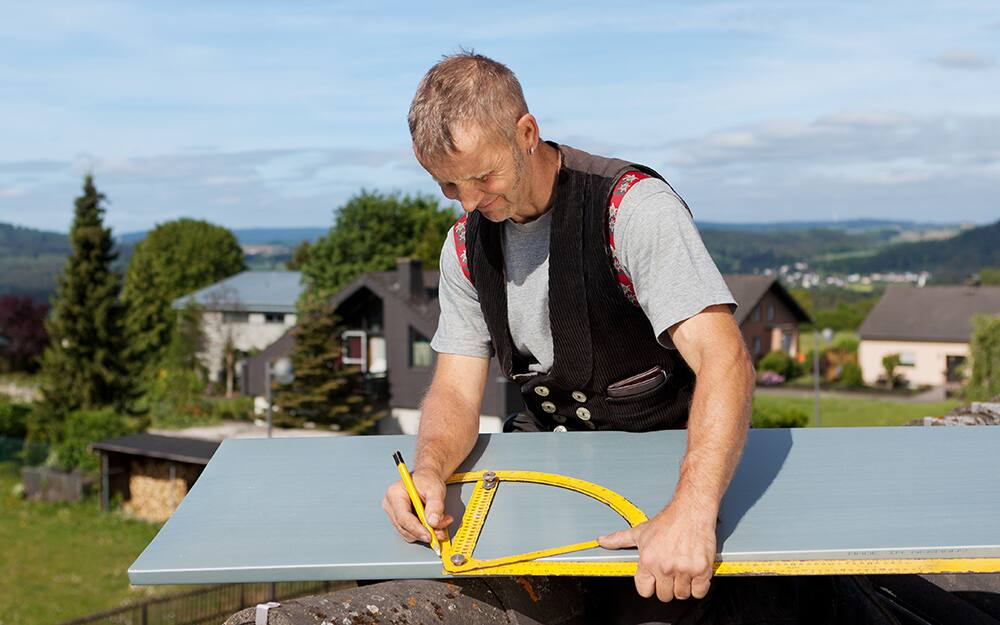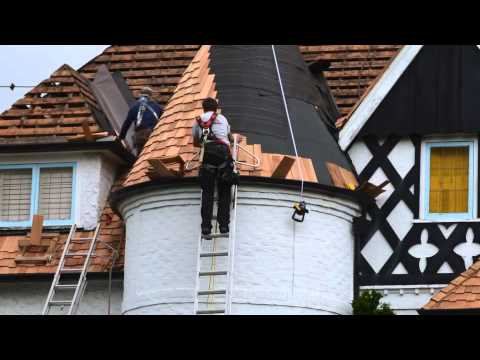### sky roof

#### How do I figure out the size of my roof?

Multiply your house length by your house width to get the area. (For example, 40 feet x 30 feet = 1,200 square feet.) Next, multiply the area by your roof’s pitch. (1,200 x 1.05 = 1,260 square feet.)

#### Can you measure a roof from the ground?

If you’re planning work on a roof and don’t have the building plans, taking preliminary measurements from the ground allows you to approximate the roof area and estimate some costs. … But, you can measure a gable or flat roof from the ground with the help of an assistant.

### Confidence In Your Bids & Bottom Line.

Our aerial roof measurement reports are drafted by expert CAD technicians and reviewed by a quality assurance professional, certifying absolute data accuracy on every report. Precision is paramount at Sky Roof Measure, and that’s how we guarantee a 98% or better accuracy rate on every plane, eave, rake, ridge, hip and valley. Be confident in your bids, and submit estimates that align to your profit goals.
Sky Roof Measure provides accurate 3D satellite roof measurements, aerial roof measurements, roofing reports for USA Canada roofers companies, insurance adjusters, and more.Sky Roof Measure Accurate & Consistent

Accuracy Is The Key To Success. No Other Words Can Explain The Importance. Our Aerial Roof Measurement Reports Are Prepared By Trained Technicians Followed By A 3 Step Verification Process By A Quality Assurance Professional, Certifying Absolute Data Accuracy On Every Report We Deliver.

Fastest Delivery Turnaround

Proven And Consistent Fastest Delivery Turnaround In The Industry. Measurements Can Be Provided In As Low As 3 hours*

Gain Competitive Edge

A Wise Man Once Said, “When You Arrive Empty Handed, You’ll Return Empty Handed”. Order A Report A Couple Of Hours Ahead Of Your Schedule And Arrive Confident With All The Data In Your Hand. Spend More Face Time With Your Client And Waste No Time Just Taking Pictures, Uploading And Waiting Endlessly.Exactly What You Need

Our Aerial Roof Measurement Reports Are Comprehensive, Easy To Use, And Include All Information You’ll Ever Need. Be Confident In Your Bids, And Submit Estimates That Align To Your Bottom Line.

### How To Measure The Roof Using Google Earth

There are many great benefits to using Google Earth to measure your roof but nothing is as good as using DIY’s satellite roof measurement. The first of which is that you don’t even have to go up on the roof. This can make it even safer to do your measuring and it also removes a level of guessing to your calculation.

To begin with, you’re going to go to the app on your mobile device, or you will need to go to Google Earth on your laptop or desktop computer. There are many options here and you’ll want to select the one that allows you to enter the address. You can also zoom the map and click on the starting point of the roof you wish to select. After you’ve done this, you can click along the outer edge of the roof that you wish to calculate the area for.

# How To Measure A Roof

Getting accurate roof measurements can be difficult but with this roofing calculator, you’ll be well prepared to take measurements of any roof. Whether you’re a contractor looking to train a new employee or a homeowner looking to do some renovations, you’ll find this guide helpful for any roofing project. Use the step-by-step guide below to accurately measure your roof and estimate roofing costs.## Satellite Roof Measurements: Features of a Roof Report

• Accurate Roof Pitch Measurements
• Roof Measurements including AreaRakesRidgesHips and Valleys, and every edge is separately measured and identified.
• CAD style drawings with colored lines identifying every type of roof edge
• Multiple image providers for over 98% coverage of the USA & Canada
• Also supports file formats such as XML,RXF,DXF/DWG AND WRL

### MEASURING THE PITCHTo calculate the area of your roof, first you’ll need to calculate the pitch of it:

1. First, use your measuring tape to measure 12 inches on your large level and make a mark at the 12-inch line.
3. Climb to the top of your roof.
4. After that, place your level against the gable trim and flat against the side of the house.
5. Using your measuring tape, measure from the 12-inch mark on your level up to the bottom edge of your gable trim. This number is your rise.
6. Each rise measurement is written as “# in 12” and this is your roof’s pitch. (For example, if your rise is 4 inches, your roof’s pitch is 4 in 12.)

### CALCULATING THE AREAIf you want to get the exact area measurement for your roof, you can follow these steps:

1. Once you have your roof’s pitch, divide the number by 12. (For example, if your roof’s pitch is 4 in 12, you would divide 4 by 12. This would yield 1/3.)
2. Next, square your result. (If your number is 1/3, squared would yield 1/9.)
3. After that, add 1 to your number. (1/9 + 1 = 10/9.)
4. Next, figure out the square root of your new number. (The square root of 10/9 is 1.05.)
5. Next, use your measuring tape to measure the length of your house. (Be sure to include overhangs.)
6. After that, measure the width of your house. (Be sure to include overhangs.)
7. Multiply your house length by your house width to get the area. (For example, 40 feet x 30 feet = 1,200 square feet.)
8. Next, multiply the area by your roof’s pitch. (1,200 x 1.05 = 1,260 square feet.)
9. To allow for hips, ridges, and waste, add 10% of your final number for a gable roof and 17% of your final number for a cottage roof. (Your total number would be either 1,386 or 1,474 square feet.)

## Acreage Calculator – Measure the area of a plot of land

The easiest way to measure the acreage of a plot of land is to start by entering an address that is associated with the plot of land you need the area of. In rural areas where an address may not be available, you can enter the cross street or even the GPS coordinate of a point on the land. For GPS coordinates be sure to enter the latitude followed a comma then the longitude ex. (41.87811, -87.629798). In any case this will place a marker that you can use as a reference point to draw the area on the map. Once you are finished drawing the area calculator will display the area of the shape above the map.## How to use the google maps area calculator tool to measure a roof

Special precautions must be taken when measuring the area of a roof. The images that appear on google maps are often at a slight angle, which combined with the angle of a roof can cause errors. For this reason it is best to outline the roof at points which are all at the same elevation. On a simple house for instance you would only click on the corners of the roof and not on any points along the peak as that would cause an error. After measuring the area of the footprint you can estimate the actual roof area based on the angle of the roof. Similarly if you using the area calculator tool to measure a roof with multiple levels, you should do the individual section separately. The google maps area calculator is not 100% accurate. Do not rely on this tool as your only resource in making important decisions.

### How to Measure a Quonset Roof

1. Measure the horizontal width of the Quonset roof to determine the diameter. …
2. Multiply the diameter dimension time 3.14, referred to as “pi,” and then divide the result by 2 to determine the circumference measurement of the Quonset roof.# How to Measure the Roof of a Round Building

1 . Measure the outer diameter of the building.

2 . Measure the amount of horizontal overhang between the building’s outer wall and the roof’s outer edge.

3 . Multiply the amount of overhang by two to include the opposite side of the building.

4 . Add the total overhang to the diameter. For example, a building diameter of 20 feet and an overhang of 1 foot on one side results in a total roof diameter of 22 feet.

5 . Measure the length of the rafters from the peak to the lower edge.

6. Calculate the total surface area of the roof using the following formula: pi times the radius times the rafter length, also called the slant height. To find the surface area using the diameter in the previous example and a rafter length of 20 feet, use the following calculations and use 3.14 as an approximation for pi:

Radius = 22 / 2 = 11.

Surface area in square feet = 3.14 * 11 * 20 = 690.8.

7 .  Determine the vertical height of the roof by finding the square root of the result of subtracting the squares of the rafter length and the radius. Using the previous results, the answer would look like this:

Rafter length squared = 20 * 20 = 400.

Radius squared = 11 * 11 = 121.

So 400 – 121 = 279.

The square root of 279 is approximately 16.7. In this example, the vertical rise of the roof is 16.7 feet from the outer edge to the peak of the roof.# Measuring Roof Slope and Pitch

### Slope

Slope is the incline of the roof expressed as a ratio of the vertical rise to the horizontal run, where the run is some portion of the span. This ratio is always expressed as inches per foot.

### Slope Ratio

A roof that rises 4 inches for every 1 foot or 12 inches of run is said to have a “4 in 12” slope. If the rise is 6 inches for every 12 inches of run, then the roof slope is “6 in 12.”

The slope can be expressed numerically as a ratio. The slope ratio represents a certain amount of vertical rise for every 12 inches of horizontal run. For example, a “4 in 12” slope can be expressed as the ratio of 4:12. A “6 in 12” slope is expressed as 6:12.

### Pitch

Pitch is the incline of the roof expressed as a fraction derived by dividing the rise by the span, where the roof span is the distance between the outside of one wall’s top plate to another.

### Pitch Fraction

Historically the word “pitch” meant a ratio between the ridge height to the entire span/width of the building or ratio between the rafter length to the building width. And back then, the ridge was typically in the middle of the span. This is no longer the case in modern building practices. The ridge can be placed anywhere in the span, from directly middle to either span endpoint.
A roof that rises 8 feet over a 24-foot span was said to have a “1 to 3” pitch. If the rise is 4 feet over a 24-foot span, then the roof pitch was said to be “1 to 6.”

The pitch can be expressed numerically as a fraction. The pitch fraction represents a certain amount of vertical rise over the entire span. For example, given a roof with a rise of 4 feet and a span of 24 feet, the pitch is “1 to 6” pitch, which can be expressed as the fraction of 1/6. A “12 to 24” pitch is expressed as 1/2.